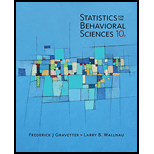# It appears that there is some truth to the old adage ‘That which doesn’t kill us makes us stronger.” Seery, Holman, and Silver (2010) found that individuals with some history of adversity report better mental health and higher well-being compared to people with little or no history of adversity. In an attempt to examine this phenomenon, a researcher surveys a group of college students to determine the negative life events that they experienced in the past 5 years and their current feeling of well-being. For n = 18 participants with 2 or fewer negative experiences, the average well-being score is M = 42 with S S = 398 , and for n = 16 participants with 5 to 10 negative experiences the average score is M = 48.6 with S S = 370 . a. Is there a significant difference between the two populations represented by these two samples? Use a two-tailed test with α = .01 . b. Compute Cohen’s d to measure the size of the effect. c. Write a sentence demonstrating how the outcome of the hypothesis test and the measure of effect size would appear in a research report.### Statistics for The Behavioral Scie...

10th Edition
Frederick J Gravetter + 1 other
Publisher: Cengage Learning
ISBN: 9781305504912### Statistics for The Behavioral Scie...

10th Edition
Frederick J Gravetter + 1 other
Publisher: Cengage Learning
ISBN: 9781305504912

#### Solutions

Chapter
Section
Chapter 10, Problem 8P
Textbook Problem

## Expert Solution

### Want to see the full answer?

Check out a sample textbook solution.See solution

### Want to see this answer and more?

Experts are waiting 24/7 to provide step-by-step solutions in as fast as 30 minutes!*

See Solution

*Response times vary by subject and question complexity. Median response time is 34 minutes and may be longer for new subjects.

Find more solutions based on key concepts
Show solutions
In Exercises 4756, solve the given equation for the indicated variable. 27=32x1

Finite Mathematics and Applied Calculus (MindTap Course List)

Calculate y'. 37. y=sin(tan1+x3)

Single Variable Calculus: Early Transcendentals, Volume I

Finding Limits In Exercises 93-110, find the limit (if it exists). limx6x4

Calculus: An Applied Approach (MindTap Course List)

In Exercises 14, find the values of x that satisfy the inequality (inequalities). 2. 2 3x + 1 7

Applied Calculus for the Managerial, Life, and Social Sciences: A Brief Approach

17. Find the general solution of the separable differential equation .

Mathematical Applications for the Management, Life, and Social Sciences

Combine the following complex numbers. (7+2i)+(34i)

Trigonometry (MindTap Course List)

Find the limit or show that it does not exist. limx[ln(1+x2)ln(1+x)]

Single Variable Calculus: Early Transcendentals

One of the vector forms of Green’s Theorem says that a) b) c) d)

Study Guide for Stewart's Multivariable Calculus, 8th

For f(x)=x2+x, we may write f (x) = (h g)(x), where: a) h(x)=x and g(x) = x2 + x b) h(x) = x2 + x and g(x)=x c...

Study Guide for Stewart's Single Variable Calculus: Early Transcendentals, 8th

Mathematics For Machine Technology

A Bag of coins A bag contains 30 coins, some dimes and some quarters. The total amount of money in the bag is 3...

Functions and Change: A Modeling Approach to College Algebra (MindTap Course List)

Consider the following data for two variables, x and y. Compute the standardized residuals for these data. Do ...

Modern Business Statistics with Microsoft Office Excel (with XLSTAT Education Edition Printed Access Card) (MindTap Course List)

In problems 2126 solve the given intial-value problem. 23. (4y + 2t 5) dt + (6y + 4t 1) dy = 0, y(1) = 2

A First Course in Differential Equations with Modeling Applications (MindTap Course List)